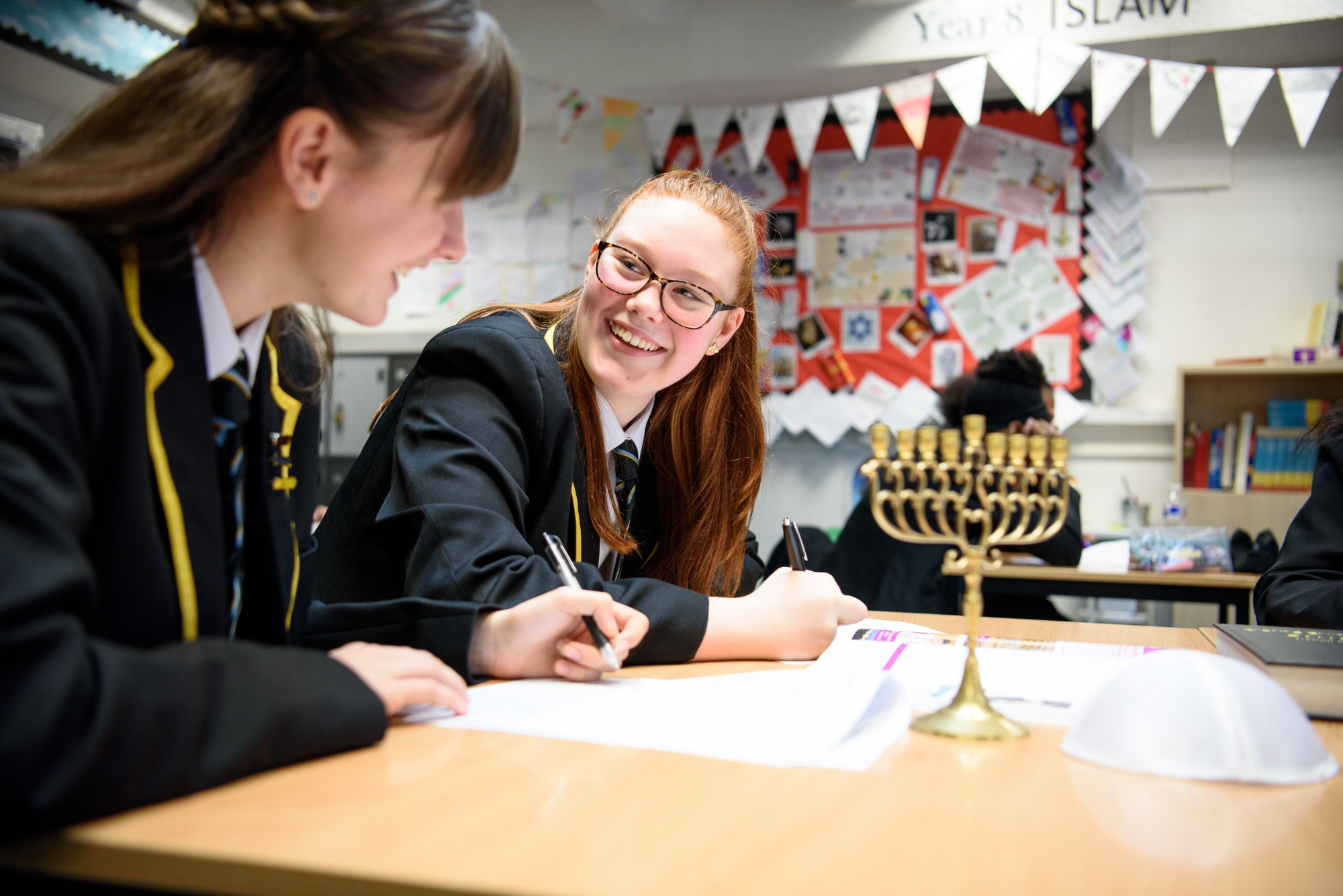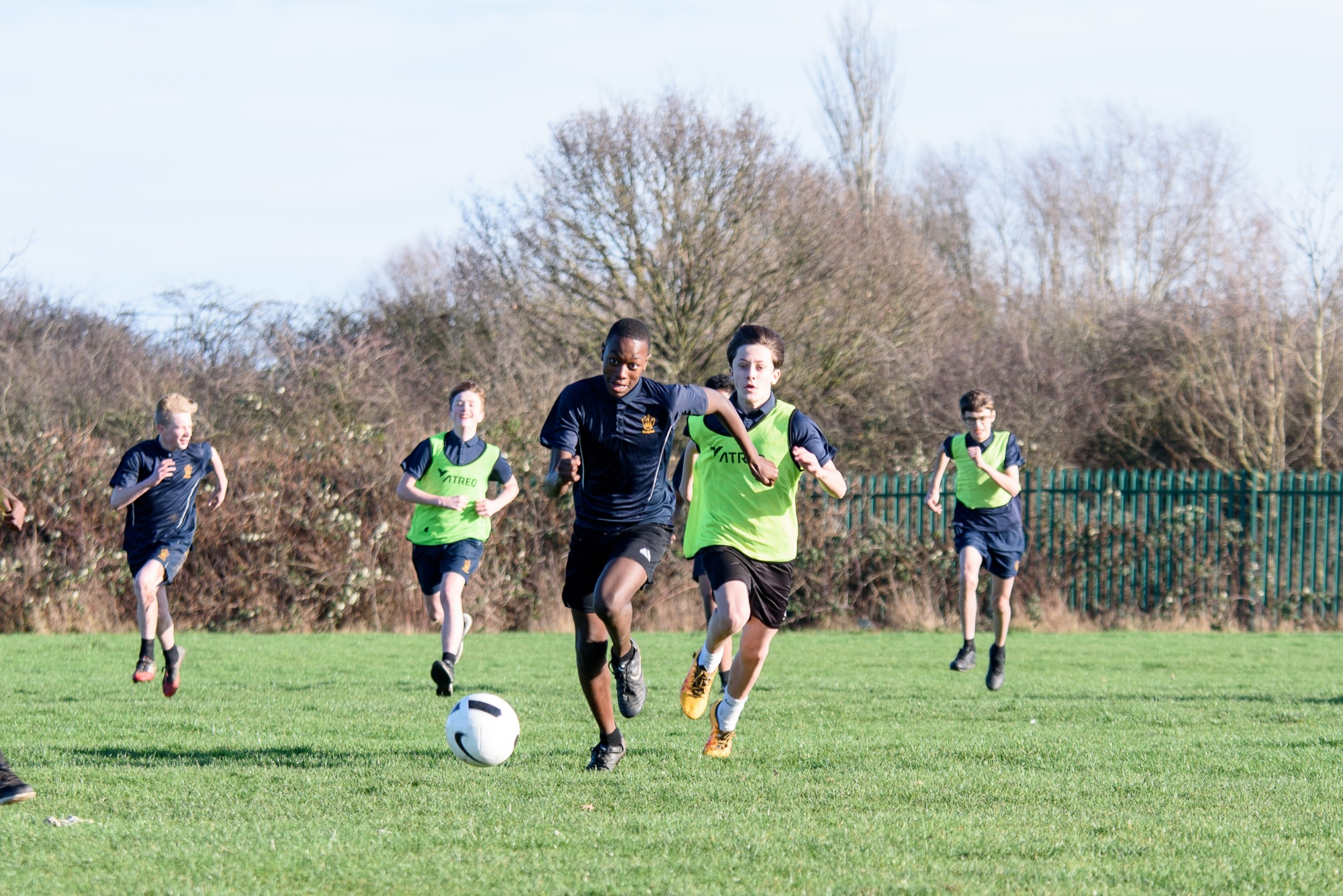•••••# Mathematics

## Welcome to Mathematics

The Maths department at De La Salle has a long history of success, achieving above the national average results at GCSE for many years, enabling students to make outstanding progress.

Mathematics is a creative and highly inter-connected discipline that has been developed over centuries, providing the solution to some of history’s most intriguing problems. It is essential to everyday life, critical to science, technology and engineering, and necessary for financial literacy and most forms of employment. A high-quality mathematics education therefore provides a foundation for understanding the world, the ability to reason mathematically, an appreciation of the beauty and power of mathematics, and a sense of enjoyment and curiosity about the subject.

The national curriculum for mathematics aims to ensure that all pupils:

• become fluent in the fundamentals of mathematics, including through varied and frequent practice with increasingly complex problems over time, so that pupils develop conceptual understanding and the ability to recall and apply knowledge rapidly and accurately.
• reason mathematically by following a line of enquiry, conjecturing relationships and generalisations, and developing an argument, justification or proof using mathematical language
• can solve problems by applying their mathematics to a variety of routine and non-routine problems with increasing sophistication, including breaking down problems into a series of simpler steps and persevering in seeking solutions.

Mathematics is split into five areas which are as follows:

• Number
• Algebra
• Ratio and Proportion
• Geometry and Measures
• Probability and Statistics

### KS3 Mathematics

In Years 7 & 8, students are taught in the same set for all their subjects. Year 7 and 8 are taught using the WhiteRose schemes of learning and resources which encourage mathematical reasoning and help develop mastery in mathematics.  All students follow the same scheme of learning and have six mathematics lessons across a two-week timetable.

Home learning is set at least once per week and students sit two assessments in class, twice each half term.  Students keep their own gap analysis (identified gaps in learning) inside their mathematics books available for parent/guardians to see.  There is a tracker which monitors grades over time at the front of their books.

### KS4 Mathematics

Mathematics is a core subject and all students are required to follow the three-year course which commences in year 9.  The GCSE examination (Edexcel – specification 1MA1), consisting of three papers is sat by students at the end of year 11.

In KS4, students are set according to mathematics ability and have seven lessons across a two-week timetable.  Sets 1 and 2 follow the higher schemes of learning.  Sets 2 and 3 follow the intermediate schemes of learning and set 4 and 5 following the foundation schemes of learning.

All students are assessed using practice GCSE papers twice each half-term for bespoke tracking.  Students keep their own gap analysis (identified gaps in learning) inside their mathematics books available for parent/guardian to see.  There is a tracker which monitors grades over time at the front of their books.

Home learning is set at least once per week.

GCSE Mathematics is examined by three written papers taken at the end of Year 11.  The first is a non-calculator paper, followed by two calculator papers.  The time allocated for each paper is 90 minutes.

Students who sit the foundation tier can achieve grades 1 – 5 and students who sit the higher tier can achieve grades 4 - 9.

Recommended Resources

Specialist Equipment

Students are expected to bring the following equipment to school, along with the standard equipment (black pen, red pen, pencil, ruler, eraser, pencil sharpener):

• A scientific calculator (the Casio fx is recommended)
• Protractor
• A pair of compasses